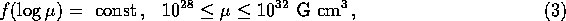Next: Other parameters of the Up: The Model Previous: Initial binary parameters

## Initial parameters of compact stars

As we only deal with initially massive binary systems (their primary's mass is higher than 10 ), which are capable of evolving off the main sequence during less than years, the compact stars left behind stellar evolution are (in the case of the most massive stars) NSs and BHs.

We assume that a NS with a mass of is formed as result of the collapse of a star, whose core mass prior to collapse was . This corresponds to an initial mass range , taking into account that a massive star can lose more than of its initial mass during the evolution with a strong stellar wind (de Jager 1980).

We also take into account that the collapse of a massive star into a NS can be asymmetrical, so that an additional kick velocity, , presumably randomly oriented in space, should be imparted to the newborn NS. In the present calculations, the kick velocity was taken to be 75 km/s (for a more detailed study of kick velocity distributions, see Lipunov et al. 1995c).

The magnetic field of a rotating NS largely defines the evolutionary stage the star would have in a binary system (Schwartzman 1970; Davidson & Ostriker 1973; Illarionov & Sunyaev 1975). For this purpose, in our calculations we use a general classification scheme for magnetized objects elaborated by Lipunov (1992).

When the secondary component in a binary overfills its Roche lobe, the rate of accretion onto the compact star can be high enough to reach the Eddington luminosity erg/s at the ; then a supercritical regime sets in (it is worth noting that not only superaccretors but superpropellers and superejectors can exist as well; see Lipunov 1992).

If a BH is formed in due course of the evolution, it can only appear as an accreting or superaccreting X-ray source; other very interesting stages such as BH + radiopulsar which may constitute a rather large fraction of all binary pulsars after a starburst are considered in Lipunov et al. (1995a).

The distribution of the newborn NSs in the initial magnetic dipole moment is taken to beand the initial rotational period of the NS is assumed to be 1 ms.

It is not clear as yet whether the NS magnetic field decays or not (for a comprehensive review, see Chanmugam 1992). Below, we assume that the magnetic fields of NSs decays exponentially on a timescale of years. A radiopulsar is assumed to be turned ``on'' until its period P has reached a ``death-line'' value, , defined from the relation , where is the dipole magnetic moment in units of G cm, and is taken in seconds.

The mass limit for NS (the Oppenheimer-Volkoff limit) is taken to be , which corresponds to a hard equation of state of the NS matter.

The most massive stars are assumed to collapse into a BH once their mass before the collapse is (which would correspond to an initial mass of the ZAMS star as high as since a substantial mass loss due to a strong stellar wind occurs for the most massive stars). The BH mass is calculated as , where the parameter is taken to be 0.3, as follows from the studies of binaries NS + BH (Lipunov et al. 1994).Next: Other parameters of the Up: The Model Previous: Initial binary parameters

Sergei B. Popov
Fri Jun 21 20:13:26 MSD 1996